# Motion With Graphs with Examples

Article Index
Motion With Graphs
Motion With Graphs (Continue)
All Pages

Velocity vs. Time Graphs

In velocity vs. time graphs, x axis is time as in the case of position vs. time graphs and y axis is velocity. We can benefit from this graph by two ways. One of them is area under the graph which gives the displacement and the slope which gives the acceleration.

1. We have talked about different kinds of motion such as, constant motion having constant velocity, accelerated motion like speeding up or slowing down. For instance, in this graph as it seen our velocity is constant, time passes but velocity does not change. What you see when you look at this graph?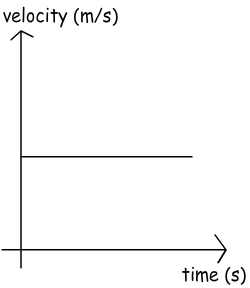We see the relation of velocity and time, how velocity is changing with time. It can be said for this graph acceleration is zero because there is no change in velocity. Moreover, from velocity vs. time graphs we can calculate displacement of the object. How can we do this? Let’s think together. First, look at the definition of displacement;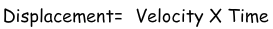Since, the velocity times time gives us displacement the area under the velocity vs. time graph also gives us the displacement of the object. Look at the example given below to understand what we mean by the area under the graph.

Example Using the given graph calculate the displacement of the object for the interval (0s – 4s).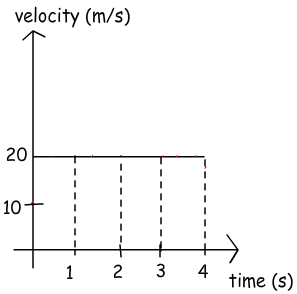To solve this problem, I use the technique given above and classic formula. The area under the graph will give us the displacement. Then we

compare the results of two techniques.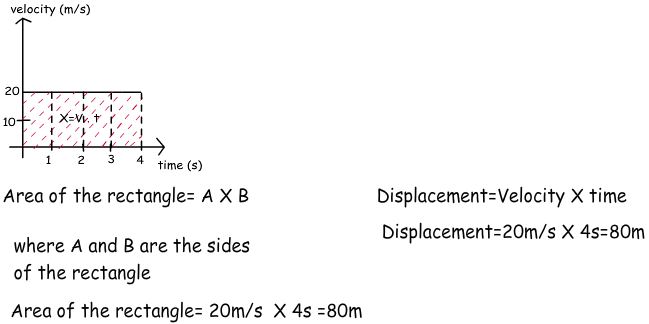As you can see, the results are the same, thus, we can say that in velocity vs. time graphs we can find the displacement by looking at the area under the graph.

2. In this graph there is a linear increase in velocity with respect to time so, the acceleration of the motion is constant. Moreover, we can also calculate the displacement by looking at under the area of the graph. Let’s solve another example for deep understanding.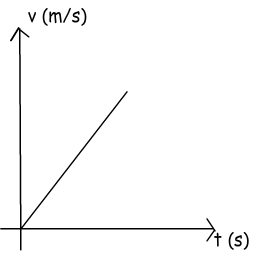Example Calculate the displacement of the car from the given graph.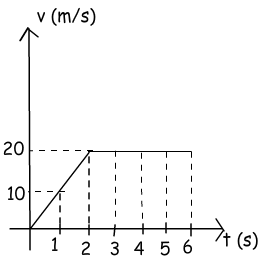We can calculate displacement by using the area under the graph, to do this we can first calculate the area of the triangle shown with blue lines and then rectangle shown with red lines. Finally the sum of these two areas gives us the total displacement of the car.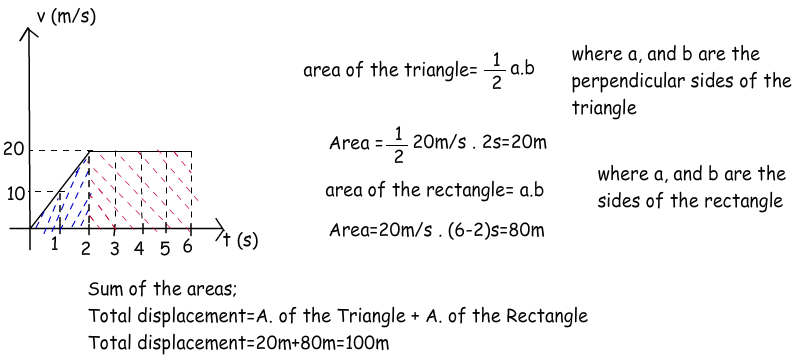3. This graph shows the different accelerated motions. The lines are curved because acceleration is not constant. They represent the decreasing and increasing velocity in positive and negative directions. However, we do not deal with such problems now.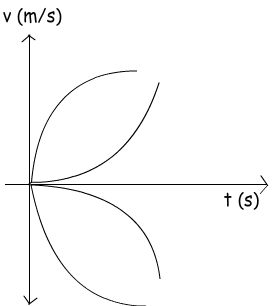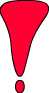We have discussed the velocity vs. time graphs. When you look at these graphs you can find the displacement and acceleration of the object by looking at the slope for acceleration and area for displacement.

Related